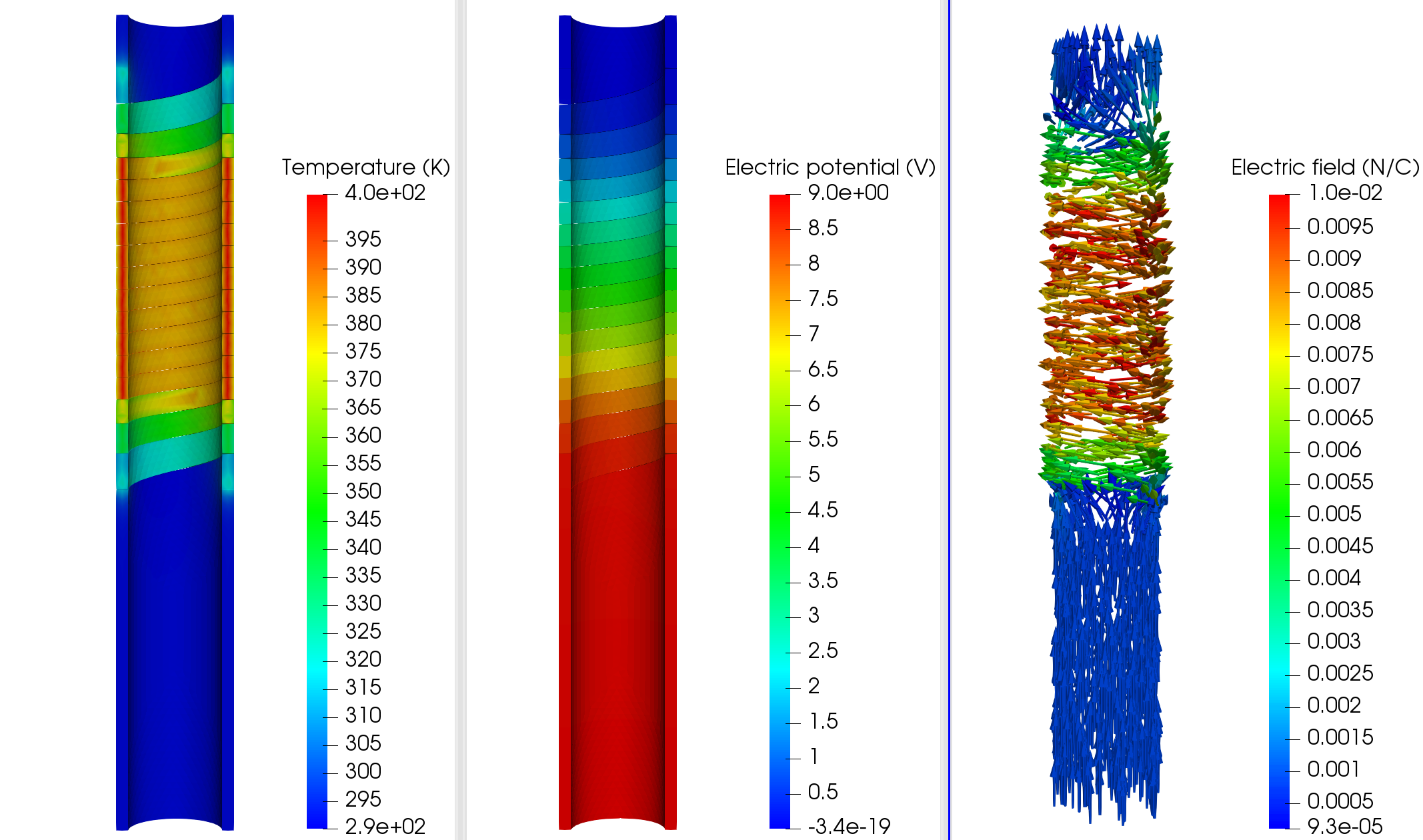# ElectroMagnet

In this example, we will estimate the rise in temperature due to Joules losses in a stranded conductor. An electrical potential $V_D$ is applied to the entry/exit of the conductor which is also water cooled.

## 1. Running the case

The command line to run this case in linear is

``mpirun -np 4 feelpp_toolbox_thermoelectric --case "github:{path:toolboxes/thermoelectric/cases/ElectroMagnets/HL-31_H1}"``
Case option
`--case "github:{path:toolboxes/thermoelectric/cases/ElectroMagnets/HL-31_H1}"`

The command line to run this case in non linear is

``mpirun -np 4 feelpp_toolbox_thermoelectric --case "github:{path:toolboxes/thermoelectric/cases/ElectroMagnets/HL-31_H1}" --case.config-file HL-31_H1_nonlinear.cfg``
Case option
`--case "github:{path:toolboxes/thermoelectric/cases/ElectroMagnets/HL-31_H1}"`
Case config file option
`--case.config-file HL-31_H1_nonlinear.cfg`

## 2. Data files

HL-31_H1 Github directory with:

The mesh can be found on Girder

## 3. Geometry

The conductor consists in a solenoid, which is one helix of a magnet.

The mesh can be retrieve from girder with the following ID: 5af59e88b0e9574027047fc0 (see girder).

## 4. Input parameters

Name Description Value Unit

$\sigma_0$

electric potential at reference temperature

53e3

$S/mm$

$V_D$

electrical potential

9

$V$

$\alpha$

temperature coefficient

3.6e-3

$K^{-1}$

L

Lorentz number

2.47e-8

$W\cdot\Omega\cdot K^{-2}$

$T_0$

reference temperature

290

$K$

h

transfer coefficient

0.085

$W\cdot m^{-2}\cdot K^{-1}$

$T_w$

water temperature

290

$K$

``````"Parameters":
{
"sigma0":53e3, //[ S/mm ]
"T0":290, //[ K ]
"alpha":3.6e-3, //[ 1/K ]
"Lorentz":2.47e-8, //[ W*Omega/(K*K) ]
"h": "0.085", //[ W/(mm^2*K) ]
"Tw": "290", //[ K ]
"VD": "9" //[ V ]
},``````

### 4.1. Model & Toolbox

• This problem is fully described by a Thermo-Electric model, namely a poisson equation for the electrical potential $V$ and a standard heat equation for the temperature field $T$ with Joules losses as a source term. Due to the dependence of the thermic and electric conductivities to the temperature, the problem is non linear. We can describe the conductivities with the following laws:

\begin{align*} \sigma(T) &= \frac{\sigma_0}{1+\alpha(T-T_0)}\\ k(T) &= \sigma(T)*L*T \end{align*}
``````"k":"sigma0*Lorentz*heat_T/(1+alpha*(heat_T-T0)):sigma0:alpha:T0:Lorentz:heat_T", //[ W/(mm*K) ]
"sigma":"sigma0/(1+alpha*(heat_T-T0))+0*heat_T:sigma0:alpha:T0:heat_T"// [S/mm ]``````
• toolbox: thermoelectric

### 4.2. Materials

Name Description Marker Value Unit

$\sigma_0$

electric conductivity

Cu

53e3

$S.m^{-1}$

### 4.3. Boundary conditions

The boundary conditions for the electrical probleme are introduced as simple Dirichlet boundary conditions for the electric potential on the entry/exit of the conductor. For the remaining faces, as no current is flowing througth these faces, we add Homogeneous Neumann conditions.

Marker Type Value

V0

Dirichlet

0

V1

Dirichlet

$V_D$

Rint, Rext, Interface, GR_1_Interface

Neumann

0

``````"electric-potential":
{
"Dirichlet":
{
"V0":
{
"expr":"0" // V_0 [ V ]
},
"V1":
{
"expr":"VD:VD"
}
}
}``````

As for the heat equation, the forced water cooling is modeled by robin boundary condition with $T_w$ the temperature of the coolant and $h$ an heat exchange coefficient.

Marker Type Value

Rint, Rext

Robin

$h(T-T_w)$

V0, V1, Interface, GR_1_Interface

Neumann

0

``````"temperature":
{
"Robin":
{
"Rint":
{
"expr1":"h:h",
"expr2":"Tw:Tw"
},
"Rext":
{
"expr1":"h:h",
"expr2":"Tw:Tw"
}
},``````

## 5. Outputs

The main fields of concern are the electric potential $V$, the temperature $T$ and the current density $\mathbf{j}$ or the electric field $\mathbf{E}$ presented in the following figure.

``````"PostProcess":
{
"use-model-name":1,
"thermo-electric":
{
"Exports":
{
"fields":["heat.temperature","electric.electric-potential","electric.electric-field","electric.current-density","heat.pid"]
}
}
}``````